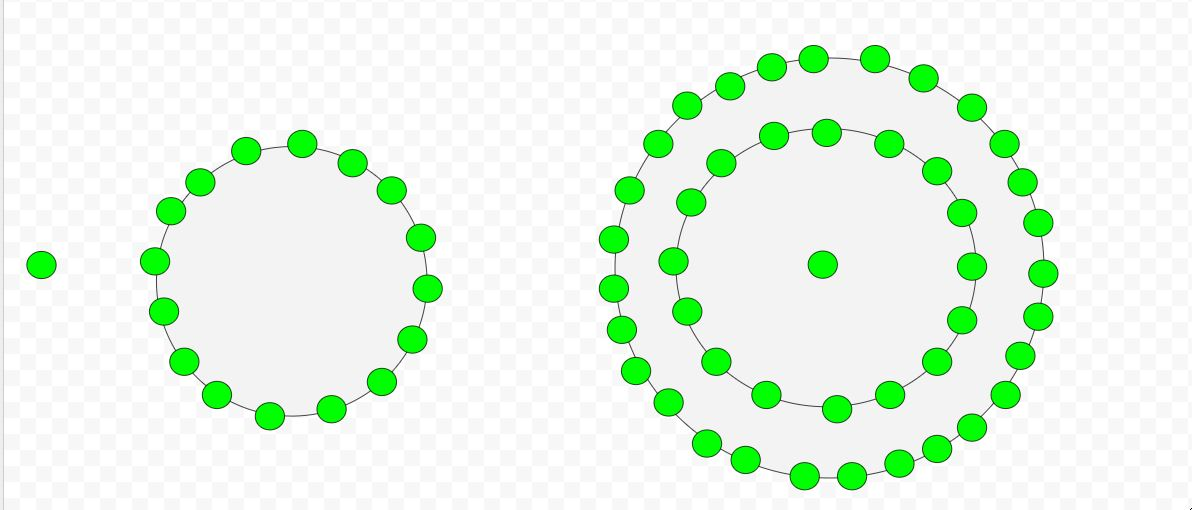Given a number n, the task is to find the nth hexadecagonal number.
A Hexadecagonal number is class of figurate number and a perfect squares. It has sixteen sided polygon called hexadecagon or hexakaidecagon. The n-th hexadecagonal number count’s the sixteen number of dots and all others dots are surrounding to its successive layer.

Examples :

Input : 2
Output :16

Input :7
Output :301## C++

 // C++ program to find Nth  // hexadecagon number  #include  using namespace std;     // Function to calculate hexadecagonal number  int hexadecagonalNum(long int n)  {      return ((14 * n * n) - 12 * n) / 2;  }     // Drivers Code  int main()  {      long int n = 5;      cout << n << "th Hexadecagonal number : ";      cout << hexadecagonalNum(n);      cout << endl;      n = 9;      cout << n << "th Hexadecagonal number : ";      cout << hexadecagonalNum(n);         return 0;  }

## Java

 // Java program to find Nth hexadecagon  // number  import java.io.*;     class GFG {         // Function to calculate hexadecagonal      // number      static long hexadecagonalNum(long n)      {          return ((14 * n * n) - 12 * n) / 2;      }             // Drivers Code      public static void main (String[] args)      {          long n = 5;          System.out.println( n + "th "           + "Hexadecagonal number : "               + hexadecagonalNum(n));                           n = 9;          System.out.println( n + "th "           + "Hexadecagonal number : "               + hexadecagonalNum(n));      }  }     // This code contribued by anuj_67.

## Python3

 # Python program to find Nth  # hexadecagon number     # Function to calculate  # hexadecagonal number  def hexadecagonalNum(n):         # Formula to calculate nth      # Centered heptagonal number      return ((14 * n * n) - 12 * n) // 2    # Driver Code  n = 5 print("%sth Hexadecagonal number : " %n,                      hexadecagonalNum(n))  n = 9 print("%sth Hexadecagonal number : " %n,                      hexadecagonalNum(n))                         # This code is contributed by ajit

## C#

 // C# program to find Nth hexadecagon  // number  using System;  class GFG {         // Function to calculate hexadecagonal      // number      static long hexadecagonalNum(long n)      {          return ((14 * n * n) - 12 * n) / 2;      }             // Drivers Code      public static void Main ()      {          long n = 5;          Console.WriteLine( n + "th "         + "Hexadecagonal number : "             + hexadecagonalNum(n));                         n = 9;          Console.WriteLine( n + "th "         + "Hexadecagonal number : "             + hexadecagonalNum(n));      }  }     // This code contribued by anuj_67.

## PHP

 

Output :

5th Hexadecagonal number : 145


Attention reader! Don’t stop learning now. Get hold of all the important DSA concepts with the DSA Self Paced Course at a student-friendly price and become industry ready.

My Personal Notes arrow_drop_upCheck out this Author's contributed articles.

If you like GeeksforGeeks and would like to contribute, you can also write an article using contribute.geeksforgeeks.org or mail your article to contribute@geeksforgeeks.org. See your article appearing on the GeeksforGeeks main page and help other Geeks.

Please Improve this article if you find anything incorrect by clicking on the "Improve Article" button below.

Improved By : vt_m, jit_t